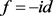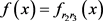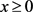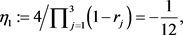﻿ 三阶迭代函数方程的反转解

# 三阶迭代函数方程的反转解Reversible Solutions of 3-rd Iterative Equation

Abstract: In this paper, we give a description of the reversible solutions for a 3-rd iterative equation. By the same method and a translational transformation, we also illustrate the reversible solutions of the related nonhomogeneous equation. Our results are illustrated by examples.

1. 引言

${f}^{k}\left(x\right)=F\left(x\right),\text{\hspace{0.17em}}\forall x\in A,$

${\lambda }_{n}{f}^{n}\left(x\right)+{\lambda }_{n-1}{f}^{n-1}\left(x\right)+\cdots +{\lambda }_{1}f\left(x\right)=F\left(x\right),\text{\hspace{0.17em}}{\lambda }_{i}\left(i=1,\cdots ,n\right)\in ℝ$

${f}^{n}\left(x\right)+{\lambda }_{n-1}{f}^{n-1}\left(x\right)+\cdots +{\lambda }_{1}f\left(x\right)+{\lambda }_{0}x=c,\text{\hspace{0.17em}}\forall x\in A,\text{\hspace{0.17em}}c\in ℝ.$ (1.1)

1974年，S. Nabeya  研究了n = 2的情况，即二阶迭代方程。2010年，张文萌与张伟年  (引理3)揭示了方程(1.1)的 ${C}^{0}$ 解与对应的齐次方程

${f}^{n}\left(x\right)+{\lambda }_{n-1}{f}^{n-1}\left(x\right)+\cdots +{\lambda }_{1}f\left(x\right)+{\lambda }_{0}x=0$ (1.2)

${C}^{0}$ 解是平移关系。将 $f\left(x\right)=rx,r\in ℂ$ 代入(1.2)，得到特征方程

$P\left(x\right):={r}^{n}+{\lambda }_{n-1}{r}^{n-1}+\cdots +{\lambda }_{1}r+{\lambda }_{0}=0,$ (1.3)

${f}^{3}\left(x\right)-\left({r}_{1}+{r}_{2}+{r}_{3}\right){f}^{2}\left(x\right)+\left({r}_{1}{r}_{2}+{r}_{1}{r}_{3}+{r}_{2}{r}_{3}\right)f\left(x\right)-{r}_{1}{r}_{2}{r}_{3}x=0,$ (1.4)

${f}^{3}\left(x\right)-\left({r}_{1}+{r}_{2}+{r}_{3}\right){f}^{2}\left(x\right)+\left({r}_{1}{r}_{2}+{r}_{1}{r}_{3}+{r}_{2}{r}_{3}\right)f\left(x\right)-{r}_{1}{r}_{2}{r}_{3}x=0$ (1.5)

2. 预备知识

$\alpha \circ f=\alpha \circ {f}^{-1}.$

$f\left(x\right)-\left({r}_{1}+{r}_{2}+{r}_{3}\right)x+\left({r}_{1}{r}_{2}+{r}_{1}{r}_{3}+{r}_{2}{r}_{3}\right)f\left(x\right)-{r}_{1}{r}_{2}{r}_{3}x=0,\text{\hspace{0.17em}}x\in ℝ,$

$\left(1+{r}_{1}{r}_{2}+{r}_{1}{r}_{3}+{r}_{2}{r}_{3}\right)f\left(x\right)=\left({r}_{1}+{r}_{2}+{r}_{3}+{r}_{1}{r}_{2}{r}_{3}\right)x,\text{\hspace{0.17em}}x\in ℝ,$ (2.1)

$f\left(x\right)={r}_{i}x,\text{\hspace{0.17em}}i=1,2,3.$

$\left({r}_{2}+{r}_{3}\right){r}_{1}^{2}={r}_{2}+{r}_{3},$

$\left({r}_{2}+{r}_{3}\right)\left({r}_{1}^{2}-1\right)=0,$

${r}_{2}+{r}_{3}\ne 0$，有 ${r}_{1}^{2}-1=0$，即 ${r}_{1}=1$ 或者 ${r}_{1}=-1$，说明 $f=id$。若 ${r}_{2}+{r}_{3}=0$，注意到 $f={f}^{-1}$，我们得到 ${r}_{1}x=\frac{1}{{r}_{1}}x$$\forall x\in ℝ$。故 ${r}_{1}^{2}-1=0$，即 $f=id$$f=-id$

3. 特征根同号

${f}^{2}\left(x\right)-\left({r}_{1}+{r}_{2}\right)f\left(x\right)+{r}_{1}{r}_{2}x=0$ (3.1)

${C}^{0}$ 解，则 $f\left(0\right)=0$，并且f严格递增，满足双边Lipschitz条件

${r}_{1}\le \left(f\left(x\right)-f\left({x}^{\prime }\right)/\left(x-{x}^{\prime }\right)\right)\le {r}_{2},\text{\hspace{0.17em}}x,{x}^{\prime }\in ℝ.$ (3.2)

i) 若f有不动点，则0是唯一不动点，且满足

$f\left(x\right):=\left\{\begin{array}{l}{f}_{i}\left(x\right),\text{\hspace{0.17em}}\text{\hspace{0.17em}}\text{\hspace{0.17em}}x\ge 0,\\ {f}_{j}\left(x\right),\text{\hspace{0.17em}}\text{ }\text{ }\text{\hspace{0.17em}}x\ge 0,\end{array}\text{\hspace{0.17em}}\text{\hspace{0.17em}}\text{\hspace{0.17em}}i,j=1,{r}_{2}{r}_{3},$ (3.3)

${f}^{2}\left(x\right)-\left({r}_{2}+{r}_{3}\right)f\left(x\right)+{r}_{2}{r}_{3}x=0.$

ii) 若f无不动点，则 $f\left(x\right)>x$$f\left(x\right)$x\in ℝ$，可以用分段定义构造。

i) 若 ${C}^{0}$ 解f有不动点0，根据引理3.2，有

$f\left(x\right):=\left\{\begin{array}{l}{r}_{1}x,\text{\hspace{0.17em}}\text{\hspace{0.17em}}\text{\hspace{0.17em}}\text{\hspace{0.17em}}\text{\hspace{0.17em}}\text{\hspace{0.17em}}\text{\hspace{0.17em}}\text{\hspace{0.17em}}\text{\hspace{0.17em}}\text{\hspace{0.17em}}x\ge 0,\\ {f}_{{r}_{2}{r}_{3}}\left(x\right),\text{\hspace{0.17em}}\text{\hspace{0.17em}}\text{\hspace{0.17em}}\text{ }x<0,\end{array}$ (3.4)

$f\left(x\right):=\left\{\begin{array}{l}{r}_{1}x,\text{\hspace{0.17em}}\text{\hspace{0.17em}}\text{\hspace{0.17em}}\text{\hspace{0.17em}}\text{\hspace{0.17em}}\text{\hspace{0.17em}}\text{\hspace{0.17em}}\text{\hspace{0.17em}}\text{\hspace{0.17em}}\text{ }\text{ }x<0,\\ {f}_{{r}_{2}{r}_{3}}\left(x\right),\text{\hspace{0.17em}}\text{\hspace{0.17em}}\text{\hspace{0.17em}}x\ge 0,\end{array}$ (3.5)

$f\left(x\right)={r}_{1}x$ 或者，其中 $x\in ℝ$

ii) 若 ${C}^{0}$ 解f无不动点，则f是对称的，依据引理2.4知f是反转解。

${f}^{3}\left(x\right)-4{f}^{2}\left(x\right)+\frac{19}{4}f\left(x\right)-\frac{3}{2}x=0,$ (3.6)

${r}^{3}-4{r}^{2}+\frac{19}{4}r-\frac{3}{2}=0$

4. 特征根不同号

i) 若是方程(1.4)的严格递增解，则f是引理3.1中给出的解。

ii) 若 $f:ℝ\to ℝ$ 是方程(1.4)的严格递减解，则 $f\left(x\right)={r}_{1}x$

$f:ℝ\to ℝ$ 是方程(1.4)的严格递减解，根据引理4.1有 $f\left(x\right)={r}_{1}x$。注意到 $f\left(x\right)={r}_{1}x$ 不是对合的，因而不是反转解。

${f}^{3}\left(x\right)-6{f}^{2}\left(x\right)-f\left(x\right)+30x=0,$ (4.1)

${r}^{3}-6{r}^{2}-r+30=0$

${r}_{1}:=-2,\text{\hspace{0.17em}}{r}_{2}:=3,\text{\hspace{0.17em}}{r}_{2}:=3.$

i) 若 $f:ℝ\to ℝ$ 是方程(3.1)的 ${C}^{0}$ 解，则f严格递增。若f有不动点，则时， $f\left(x\right)={r}_{i}x$；当 $x<0$ 时， $f\left(x\right)={r}_{j}x$，其中 $i,j=1,2$

ii) 方程(3.1)的每一个无不动点的 ${C}^{0}$$f:ℝ\to ℝ$ 由任意的初始函数确定。

i) 若是方程(1.4)的严格递增解，则f由引理4.2给出。

ii) 若 $f:ℝ\to ℝ$ 是方程(1.4)的严格递减解，则 $f\left(x\right)={r}_{1}x$

i) 方程(1.4)除 $f\left(x\right)={r}_{1}x,\text{\hspace{0.17em}}i=2,3$ 之外的所有严格递增的解都是反转解，并且可以由引理4.2构造。

ii) 方程(1.4)的唯一严格递减解 $f\left(x\right)={r}_{1}x$ 不是反转解。

$f\left(x\right):=\left\{\begin{array}{l}{r}_{2}x,\text{\hspace{0.17em}}\text{\hspace{0.17em}}\text{\hspace{0.17em}}x\ge 0,\\ {r}_{3}x,\text{\hspace{0.17em}}\text{\hspace{0.17em}}\text{\hspace{0.17em}}x<0,\end{array}$ (4.2)

$f\left(x\right):=\left\{\begin{array}{l}{r}_{2}x,\text{\hspace{0.17em}}\text{\hspace{0.17em}}\text{\hspace{0.17em}}x<0,\\ {r}_{3}x,\text{\hspace{0.17em}}\text{\hspace{0.17em}}\text{\hspace{0.17em}}x\ge 0,\end{array}$ (4.3)

$f\left(x\right)={r}_{2}x$$f\left(x\right)={r}_{3}x$，其中 $x\in ℝ$。函数(4.2)和(4.3)是对称的，然而 $f\left(x\right)={r}_{i}x,\text{\hspace{0.17em}}i=2,3$ 是不对称的，因此(4.2)和(4.3)是反转解，而 $f\left(x\right)={r}_{i}x,\text{\hspace{0.17em}}i=2,3$ 不是反转解。

ii) 如果f是方程(1.4)的 ${C}^{0}$ 严格递减解，引理4.3说明 $f\left(x\right)={r}_{1}x$。因为 $f\left(x\right)={r}_{1}x$ 不是对合的，所以 $f\left(x\right)={r}_{1}x$ 不是反转解。

${r}_{1}\le \frac{f\left(x\right)-f\left({x}^{\prime }\right)}{\left(x-{x}^{\prime }\right)}\le {r}_{2}$${r}_{1},{r}_{2}\in ℝ$${r}_{1}\ne {r}_{2}.$

i) 若 $f:ℝ\to ℝ$ 是方程(1.4)的严格递增解，则 $f\left(x\right)=c$

ii) 如果 $f:ℝ\to ℝ$ 是方程(1.4)的严格减解，则f由引理4.4给出。

i) 若 $f:ℝ\to ℝ$ 是方程(1.4)的严格递增解，则 $f\left(x\right)={r}_{3}x$

ii) 若 $f:ℝ\to ℝ$ 是方程(1.4)的严格递减解，则 $f\left(x\right)={r}_{1}x$$f\left(x\right)={r}_{2}x$

5. 临界情况及进一步讨论

i) 若 $r=1$，则 $f\left(x\right)=x+c$，其中c是实常数。

ii) 若 $r=-1$，则 $f\left(x\right)=-x$

i) 若 $r=1$，则方程(1.4)的每一个 ${C}^{0}$$f\left(x\right)=x+c$ 是反转的，其中c是实常数。

ii) 若 $r=-1$，则唯一的 ${C}^{0}$$f\left(x\right)=-x$ 是反转的。

ii) 若 $r=-1$，引理5.1说明 $f\left(x\right)=-x$ 是方程(1.4)的唯一的 ${C}^{0}$ 解。引理2.3和引理2.5说明 $f\left(x\right)=-x$ 是反转的。

$g\left(x\right)=f\left(x+\eta \right)-\eta ,\text{\hspace{0.17em}}\eta :=c/{\prod }_{j=1}^{n}\left(1-{r}_{j}\right),$

${g}^{n}\left(x\right)+{\lambda }_{n-1}{g}^{n-1}\left(x\right)+\cdots +{\lambda }_{1}g\left(x\right)+{\lambda }_{0}x=0,$

$f\left(x\right)=g\left(x-\eta \right)+\eta ,\text{\hspace{0.17em}}x\in ℝ,$ (5.1)

${f}^{3}\left(x\right)-19f\left(x\right)-30x=0,$ (5.2)

${g}^{3}\left(x\right)-19g\left(x\right)-30x=0$

$-3\le \frac{{g}_{d}\left(x\right)-{g}_{d}\left({x}^{\prime }\right)}{\left(x-{x}^{\prime }\right)}\le -2,\text{\hspace{0.17em}}x\ne {x}^{\prime }.$ (5.3)${f}_{3}\left(x\right):={g}_{3}\left(x-{\eta }_{1}\right)+{\eta }_{1}=5\left(x+\frac{1}{12}\right)-\frac{1}{12}=5x+\frac{1}{3}$

${f}^{3}\left(x\right)+3{f}^{2}\left(x\right)+3f\left(x\right)+x=8.$ (5.4)

${r}^{3}+3{r}^{2}+3r+1=0$

${g}^{3}\left(x\right)+3{g}^{2}\left(x\right)+3g\left(x\right)+x=0$

${\eta }_{2}:=8/{\prod }_{j=1}^{3}\left(1-{r}_{j}\right)=1,$

$f\left(x\right)=g\left(x-{\eta }_{2}\right)+{\eta }_{2}=-\left(x-1\right)+1=-x+2$

 Jarczyk, W. (2002) Reversible Interval Homeomorphisms. Journal of Mathematical Analysis and Applications, 272, 473-479.
https://doi.org/10.1016/S0022-247X(02)00164-6

 Jarczyk, W. (2002) Reversibility of Interval Homeomorphisms with No Fixed Points. Aequationes Mathematicae, 63, 66-75.
https://doi.org/10.1007/s00010-002-8005-9

 Babbage, C. (1815) An Essay towards the Calculus of Functions. Philosophical Transaction, 105, 389-423.
https://doi.org/10.1098/rstl.1815.0024

 Nabeya, S. (1974) On the Functional Equation f(p + qx + rf(x)) = a + bx + cf(x). Aequationes Mathematicae, 11, 199-211.
https://doi.org/10.1007/BF01834919

 Zhang, W. and Zhang, W. (2010) On Continuous Solutions of n-th Order Polynomial-Like Iterative Equations. Publicationes Mathematicae Debrecen, 76, 117-134.

 Draga, S. and Morawiec, J. (2016) Reducing the Polynomial-Like Iterative Equations Order and a Generalized Zoltan Boros’ Problem. Aequationes Mathematicae, 90, 935-950.
https://doi.org/10.1007/s00010-016-0420-4

 Draga, S. and Morawiec, J. (2015) On a Zoltan Boros’ Problem Connected with Polynomial-Like Iterative Equations. Nonlinear Analysis: Real World Applications, 26, 56-63.
https://doi.org/10.1016/j.nonrwa.2015.05.001

 Jarczyk, W. (1996) On an Equation of Linear Iteration. Aequationes Mathematicae, 51, 303-310.
https://doi.org/10.1007/BF01833285

 Liu, L. and Matkowski, J. (2018) Iterative Functional Equations and Means. Journal of Difference Equations and Applications, 24, 797-811.
https://doi.org/10.1080/10236198.2017.1356294

 Tabor, J. and Tabor, J. (1995) On a Linear Iterative Equation. Results in Mathematics, 27, 412-421.
https://doi.org/10.1007/BF03322847

 Yang, D. and Zhang, W. (2004) Characteristic Solutions of Polynomial-Like Iterative Equations. Aequationes Mathematicae, 67, 80-105.
https://doi.org/10.1007/s00010-003-2708-4

 Zhang, P. and Gong, X. (2014) Continuous Solutions of 3rd-Order Iterative Equation of Linear Dependence. Advances in Difference Equations, No. 1, 318.
https://doi.org/10.1186/1687-1847-2014-318

 Matkowski, J. and Zhang, W. (1997) Method of Characteristic for Functional Equations in Polynomial Form. Acta Mathematica Sinica, 13, 421-432.
https://doi.org/10.1007/BF02560023

Top